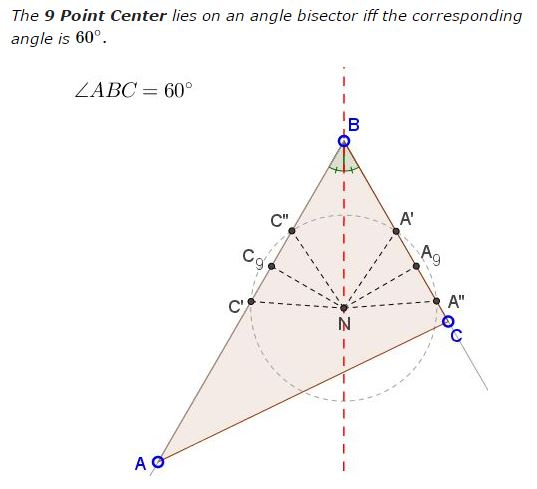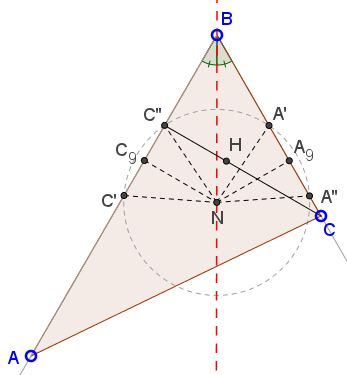# 9 Point Center on Angle Bisector

### What is this about? A Mathematical Droodle

Created with GeoGebra, 22 November, 2016

### Problem

The applet may suggest that### Solution

$\mathbf{If \;\angle B=60^{\circ}\; then\; N\in\ell_B}$

This is a consequence of the previous result: If $\angle ABC=60^{\circ}\;$ then the Euler line is perpendicular to the angle bisector: $OH\perp\ell_B.\;$ Since the circumcenter $O\;$ and the orthocenter $H\;$ are isogonal conjugate, $\Delta OBH\;$ is isosceles, and, therefore, its angle bisector from the apex is the median to the base. However, the midpoint of $HO\;$ is the 9 Point center $N,\;$ implying that $N\;$ lies on the angle bisector (of both $OBH\;$ and $ABC.$

$\mathbf{If\;N\in\ell_B\; then\; \angle B=60^{\circ}}$Define the midpoints $A',C'\;$ and the feet of the altitudes $A'',C''$ on $BC\;$ and $AB,\;$ respectively. Let $A_9\;$ and $C_9\;$ be the projections of $N\;$ on these sides. Then, since $N\in\ell_B,\;$ $NA_9=NC_9.\;$ Since the 9 Point circle passes passes through $A',C',A'',C'',\;$ $NA'=NC'=NA''=NC''.$

It follows that triangles $A_9NB\;$ and $C_9NB\;$ are equal, making the right triangles $C_9NC''\;$ and $A_9NA'\;$ also equal, such that $C''N=A'N\;$ and $\angle A_9NA''=\angle C_9NC''.\;$ From here, also, $\angle A'NB=\angle C''NB.\;$ Thus $\Delta BNA'=\Delta BNC''\;$ and, consequently, $BA'=BC''.$This means that in $\Delta CC''B,\;$ $2BC''=BC,\;$ hence $\angle CBC''=60^{\circ}.$

### Acknowledgment

The statement above has been insipred by Ignacio Larrosa Cañestro's proof of a related result.